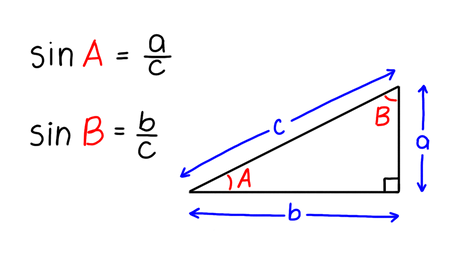VIEW IN FULL SCREEN

AQA A-level Maths Sine1. Sine
2. Values and applications
3. Right-angled traingles
4. Sine graphs and transformations

Sine of an angle is a ratio between the side opposite to the angle and the hypotenuse of a triangle.Sine is one of three trigonometric functions of an angle.sin(x) is calculated by calculating the ratio of two sides of a right-angled triangle, the opposite and the hypotenuse.The diagram shows a sine graph.1

Find the value of sin(A) by using the triangle shown.2

Find sinB if b = 5 and c = 25.3

Write down the coordinates of point A on the sine graph.4

Use your calculator to find the value of sin(60º).5

Which side of the triangle shown is the opposite?End of page